## Factorial ANOVA for Number of Colonies

The purpose of this analysis is to perform a Factorial ANOVA analysis in order to assess the effect of three independent variables (factors) which are Concentration, Cell Line and Drug on one dependent variable, which is Number of Colonies.

For the purpose of the analysis, a 4 x 2 x 3 Factorial ANOVA design will be used, with a sample size of N = 672. The following descriptive statistics are obtained: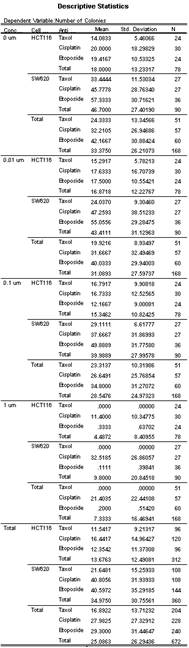The following box-plot represents the problem graphically:### Factorial ANOVA Results

The Factorial ANOVA analysis is performed with the aid of the SPSS software package. The relevant outputs are shown below.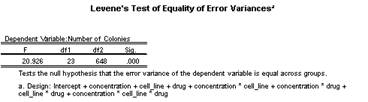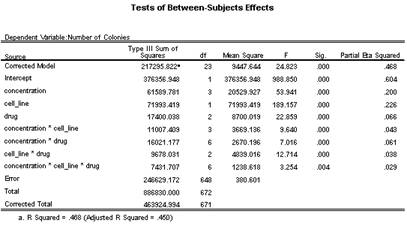The assumption of homogeneity of variances is violated, as shown by the Levene Test above, since F(23, 648) = 20.926, p < .001.

The ANOVA table above shows that all the interactions and all main factors are significant. In fact, the third order interaction Concentration*Cell Line*Drug is significant (F(6, 648) = 3.254, p = .004 < .05,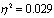). Also, the second order interaction Concentration*Cell Line is significant (F(3, 648) = 9.640, p < .001,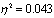), same as Concentration*Drug is significant (F(6, 648) = 7.016, p < .001,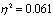) and Cell Line*Drug is significant (F(2, 648) = 12.714, p < .001,). The main effects are also significant. In fact, Concentration is significant (F(3, 648) = 53.941, p < .001,), and the same goes for Cell Line (F(1, 648) = 189.157, p < .001,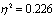) and for Drug (F(2, 648) = 22.859, p < .001,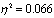).

This indicates that all the factors affect significantly the DV, and the effect of each factor on the DV depends on the level of the other factors.

The following post-hoc results are obtained: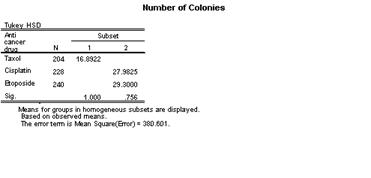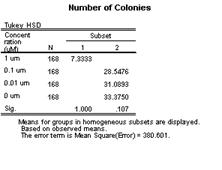### Conclusion:

The best drug is Taxol, and the best concentration is 1 um.

## Our Features

#### Customer Support

We respond quickly to questions from our customers.

#### Statistical Software

We can handle complex analyses with most of the statistical software packages available

#### Reports

We offer customized reports.

#### Free initial Consultation

We will be glad to talk to you about your needs and how we can help

#### Competitive Prices

We specialize on efficient and affordable solutions for small and medium size business

#### Call us now!

Did we mention we have a free initial consultation?

## and more...

#### Pricing

Our rates depend on the complexity of the project, and can be on an hourly basis, or on a completed project basis, depending your needs. Our rates are very competitive